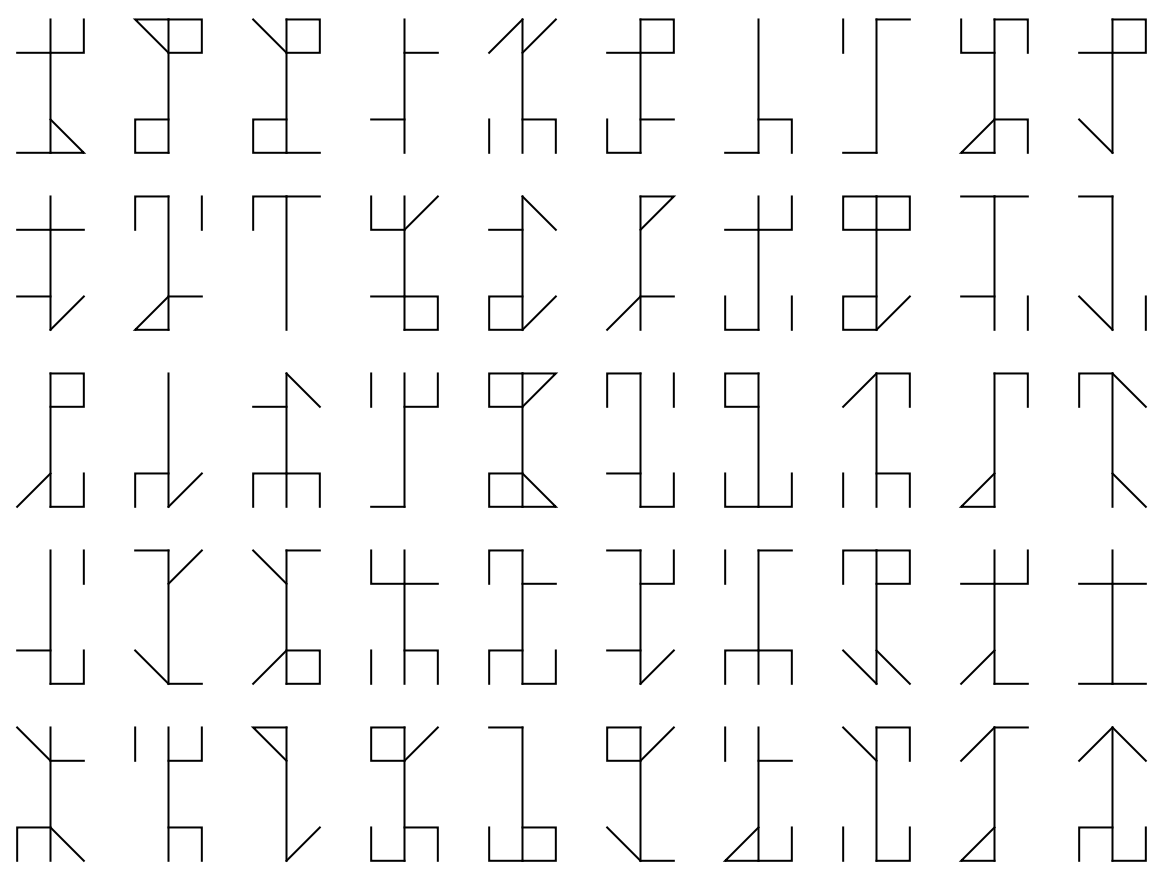#Function Repository Resource:

# CistercianNumberEncode

Encode a number according to the system devised by Cistercian monks

Contributed by: Sander Huisman
 ResourceFunction["CistercianNumberEncode"][n] encodes the number n to the Cisterian number system.

## Details and Options

Only works for integer numbers from 0 to 9999.
The output of ResourceFunction["CistercianNumberEncode"] is a Graphics object.

## Examples

### Basic Examples (1)

Encode the number 1987 to the Cistercian number system:

 In:=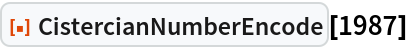Out=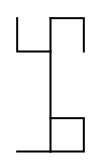### Scope (4)

Show the digits 0–9:

 In:=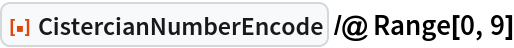Out=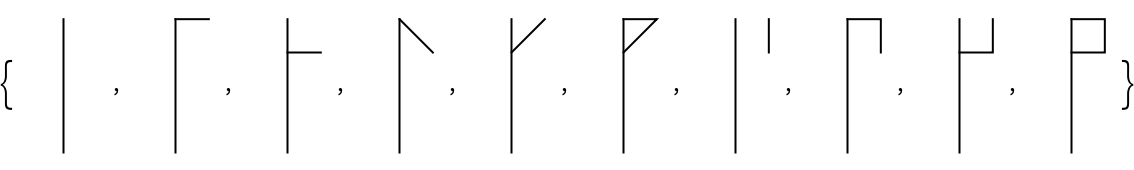Show the digits 0–90 in steps of 10:

 In:=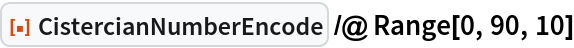Out=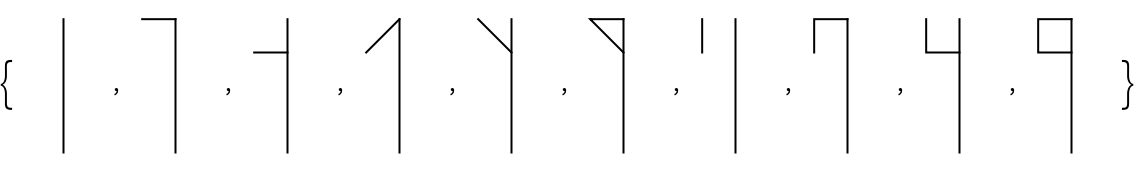Show the digits 0–900 in steps of 100:

 In:=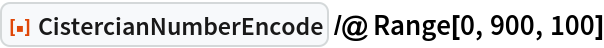Out=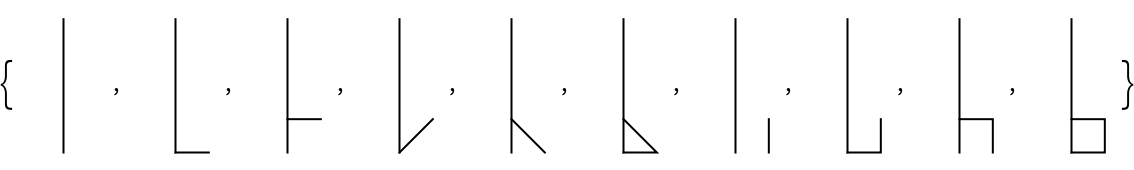Show the digits 0–9000 in steps of 1000:

 In:=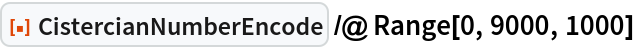Out=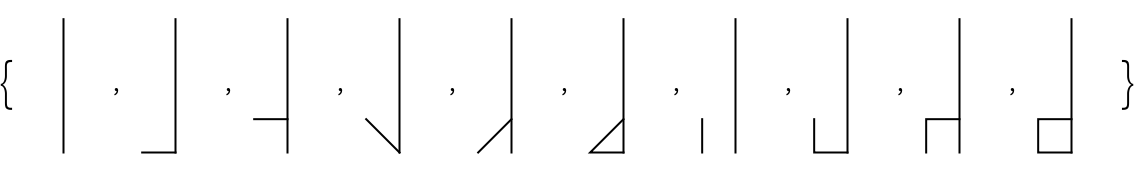### Possible Issues (3)

Negative numbers can not be encoded:

 In:=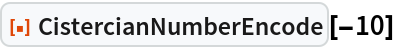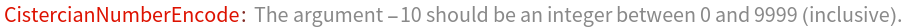Numbers larger than 9999 can not be encoded:

 In:=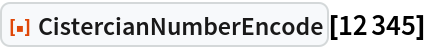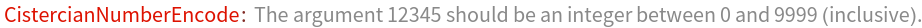Only whole numbers can be encoded:

 In:=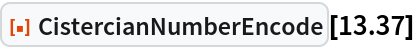Out=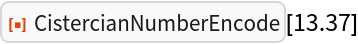### Neat Examples (2)

Encode the current year:

 In:=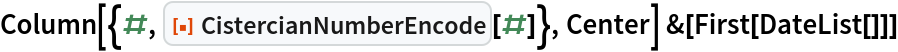Out=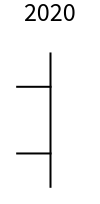Show a random collection of numbers:

 In:=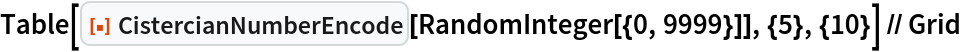Out=